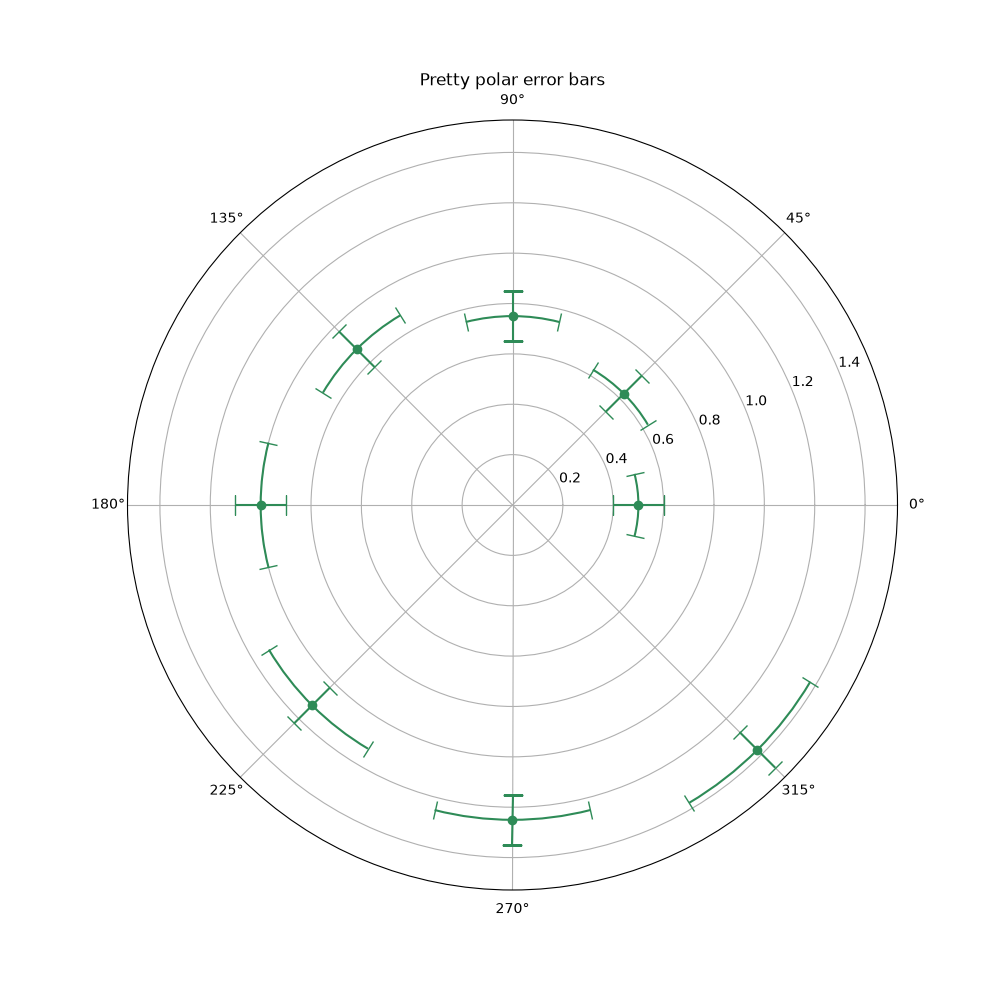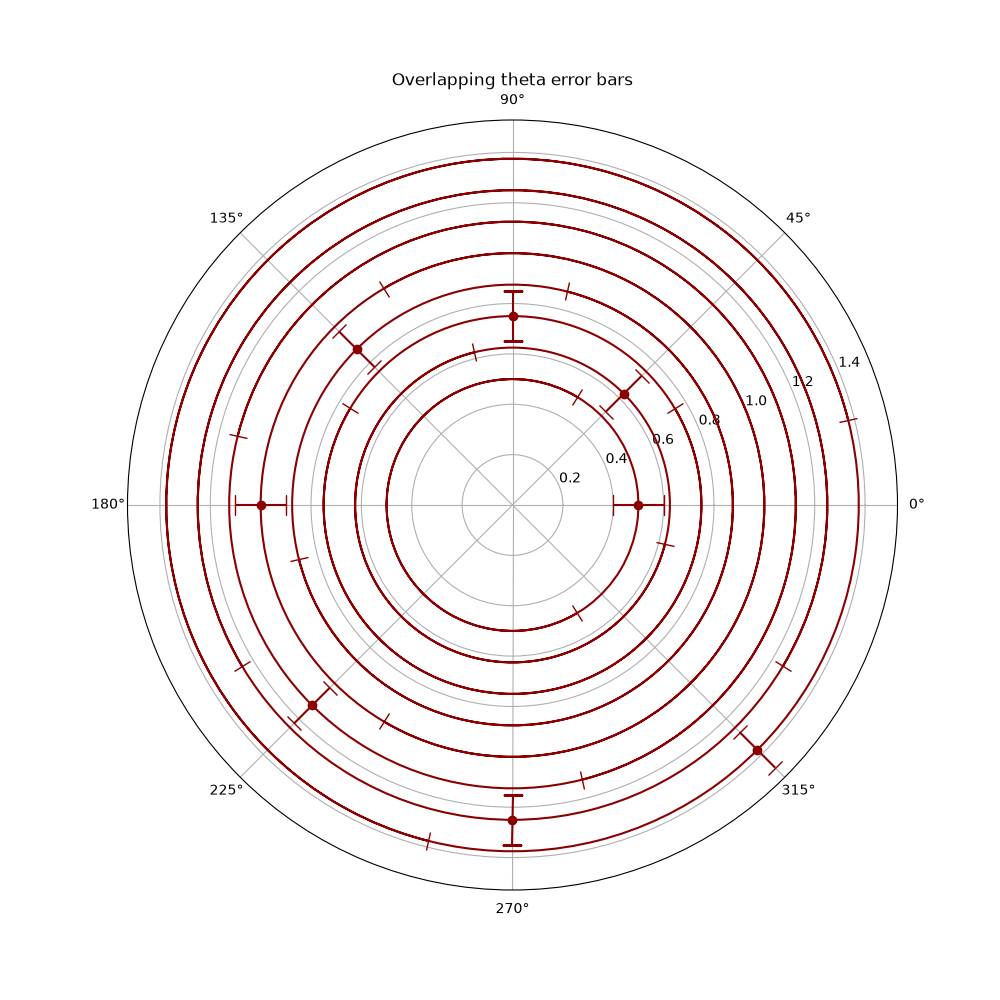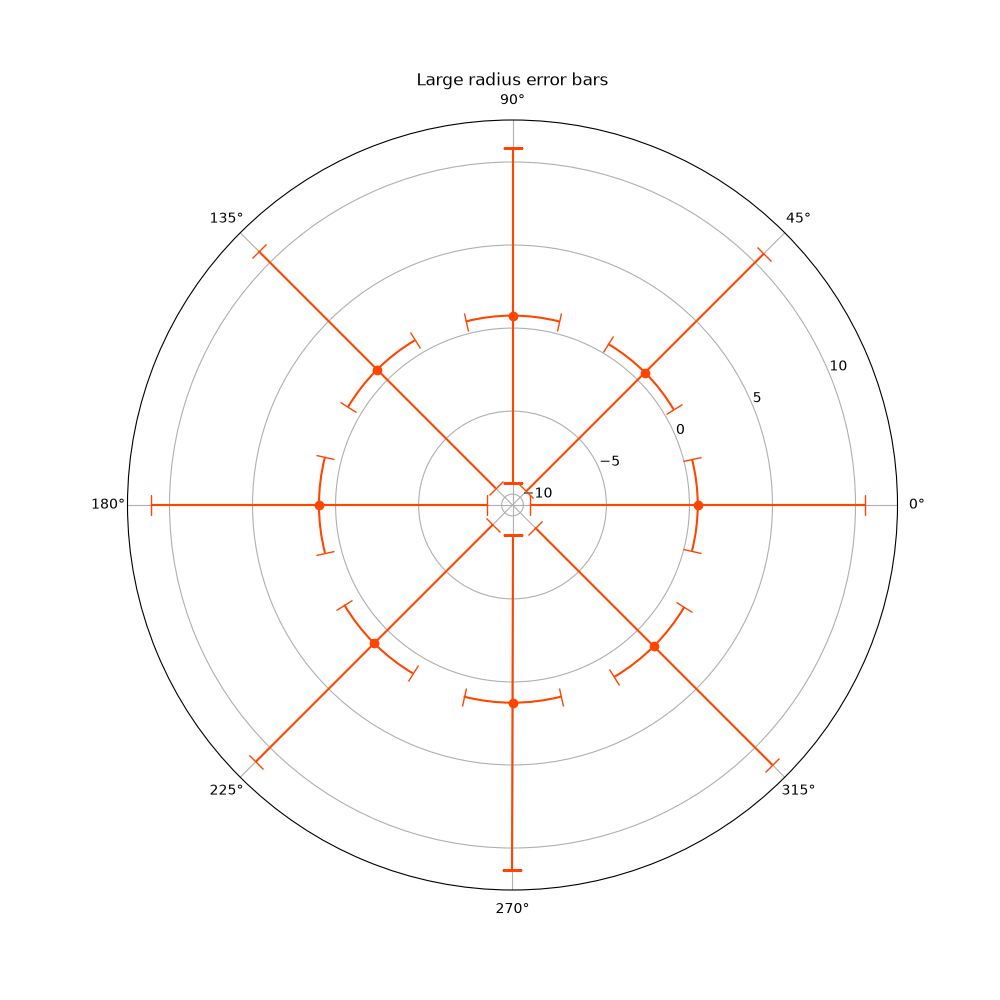# Error bar rendering on polar axis#

Demo of error bar plot in polar coordinates. Theta error bars are curved lines ended with caps oriented towards the center. Radius error bars are straight lines oriented towards center with perpendicular caps.

import numpy as np
import matplotlib.pyplot as plt

theta = np.arange(0, 2 * np.pi, np.pi / 4)
r = theta / np.pi / 2 + 0.5

fig = plt.figure(figsize=(10, 10))
ax.errorbar(theta, r, xerr=0.25, yerr=0.1, capsize=7, fmt="o", c="seagreen")
ax.set_title("Pretty polar error bars")
plt.show()Please acknowledge that large theta error bars will be overlapping. This may reduce readability of the output plot. See example figure below:

fig = plt.figure(figsize=(10, 10))
ax.errorbar(theta, r, xerr=5.25, yerr=0.1, capsize=7, fmt="o", c="darkred")
ax.set_title("Overlapping theta error bars")
plt.show()On the other hand, large radius error bars will never overlap, they just lead to unwanted scale in the data, reducing the displayed range.

fig = plt.figure(figsize=(10, 10))